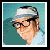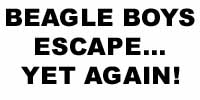Circulation: var go_t=new Date;var go_s=go_t.getTimezoneOffset(); function go_mkcd(){var s='',c='',v='',bw='',bh='',j='1.0',a=go_apn+'_'+go_apv,o=navigator.platform,r=(go_r?go_r:(go_noe?'NULL':document.referrer)); if(go_apv>=4)s=Math.round(screen.width*screen.height/100000);var uif=0;if(go_apn=='Netscape'){var i1=0,i2=0,sta;v=(navigator.javaEnabled()?'Y':'N');if(go_apv>=3)j='1.1';if(go_apv>=4){j='1.2'; c=screen.pixelDepth;bw=window.innerWidth;bh=window.innerHeight}if(go_apv>=4.06)j='1.3';if(go_apv>=5)uif=1}if(go_apn=='Microsoft Internet Explorer'){ uif=1;if(go_apv<4)r='NULL';if(go_apv>=3)v='P';if(go_apv>=4){j='1.2';v=(navigator.javaEnabled()?'Y':'N');c=screen.colorDepth} if(go_apv>=5&&navigator.platform.indexOf('Win')>=0){c=screen.colorDepth;bw=document.documentElement.offsetWidth;bh=document.documentElement.offsetHeight}} if(go_apn=='Opera'){var i1=0,i2=0,sta;var v='N'}code='' if(!go_noe)document.write(code);return code}var go_n=navigator,go_apn=go_n.appName,go_w=go_n.appVersion,go_apv,go_i,go_msie=go_w.indexOf('MSIE ') if(go_w.indexOf('Opera')>0)go_apn='Opera';if(go_msie>0){go_apv=parseInt(go_i=go_w.substring(go_msie+5));if(go_apv>3)go_apv=parseFloat(go_i)}else go_apv=parseFloat(go_w); function go_et(){window.onerror=window.oe;return true}var go_noe=false;if(navigator.userAgent.indexOf('Mac')!=-1&&navigator.userAgent.indexOf('MSIE 4')!=-1)var go_r=document.referrer;else{ window.oe=window.onerror;window.onerror=go_et;var go_r=parent.document.referrer;go_noe=go_et()}document.write(go_mkcd())*** The Comics & Stories pages have been updated *** June 19, 2003Carl Barks: 1901-2000(DP) - Local tycoon Scrooge McDuck (pictured at left) has plenty of reason to be worried... again! The notorious Beagle Boys have broken out of prison for the umpteenth time! Mr. McDuck has long been a target of the Beagle Boys, due mainly to the unbelievably large quantities of cash that he keeps on hand in his money bin on Killmotor Hill. See "Beagle Boys", page 3

Please feel free to email me with any suggestions or comments!

As I'm sure you know, all these images are the property of Disney Comics. I am not making any money off this site, I'm just doing this because I'm a big fan, so please don't sue me.